• 2021-05-25 02:52:02

实用文档

PAGE

文案大全

一元二次方程根与系数关系(韦达定理)，多元方程解法，高次方程解法

一元二次方程根与系数的关系

现行初中数学教材主要要求学生掌握一元二次方程的概念、解法及应用，而一元二次方程的根的判断式及根与系数的关系，在高中教材中的二次函数、不等式及解析几何等章节有着许多应用．本节将对一元二次方程根的判别式、根与系数的关系进行阐述．

一)、一元二次方程的根的判断式

一元二次方程，用配方法将其变形为：

(1) 当时，右端是正数．因此，方程有两个不相等的实数根：

(2) 当时，右端是零．因此，方程有两个相等的实数根：

(3) 当时，右端是负数．因此，方程没有实数根．

由于可以用的取值情况来判定一元二次方程的根的情况．因此，把叫做一元二次方程的根的判别式，表示为：

【例1】不解方程，判断下列方程的实数根的个数：

(1) (2) (3)

说明：在求判断式时，务必先把方程变形为一元二次方程的一般形式．

【例2】已知关于的一元二次方程，根据下列条件，分别求出的范围：

(1) 方程有两个不相等的实数根；(2) 方程有两个相等的实数根

(3)方程有实数根；(4) 方程无实数根．

【例3】已知实数、满足，试求、的值．

二)、一元二次方程的根与系数的关系

一元二次方程的两个根为：

所以：，

定理：如果一元二次方程的两个根为，那么：

说明：一元二次方程根与系数的关系由十六世纪的法国数学家韦达发现，所以通常把此定理称为”韦达定理”．

【例4】若是方程的两个根，试求下列各式的值：

(1) ；(2) ；(3) ；(4) ．

分析：本题若直接用求根公式求出方程的两根，再代入求值，将会出现复杂的计算．这里，可以利用韦达定理来解答．

说明：利用根与系数的关系求值，要熟练掌握以下等式变形：

*【例5】一元二次方程有两个实根，一个比3大，一个比3小，求的取值范围。

*【例6】 已知一元二次方程一个根小于0，另一根大于2，求的取值范围。

【例7】已知关于的方程，根据下列条件，分别求出的值．

(1) 方程两实根的积为5；(2) 方程的两实根满足．

分析：(1) 由韦达定理即可求之；(2) 有两种可能，一是，二是，所以要分类讨论．

解：(1) ∵方程两实根的积为5

【例8】已知是一元二次方程的两个实数根．

(1) 是否存在实数，使成立？若存在，求出的值；若不存在，请您说明理由．

(2) 求使的值为整数的实数的整数值．

说明：(1) 存在性问题的题型，通常是先假设存在，然后推导其值，若能求出，则说明存在，否则即不存在．

练习：

1．一元二次方程有两个不相等的实数根，则的取值范围是()

A．B．C．D．

2．若是方程的两个根，则的值为()

A．B．C．D．

3．已知菱形ABCD的边长为5，两条对角线交于O点，且OA、OB的长分别是关于的方程的根，则等于()

A．B．C．D．

4．若实数，且满足，则的值为

()

A．B．C．D．

5．若方程的两根之差为1，则的值是 _____ ．

6．设是方程的两实根，是关于的方程的两实根，则= _____ ，= _____ ．

7．对于二次三项式，小明得出如下结论：无论取什么实数，其值都不可能等于10，您是否同意他的看法？请您说明理由．

*8．一元二次方程两根、满足

求取值范围。

9．已知关于的一元二次方程．

(1) 求证：不论为任何实数，方程总有两个不相等的实数根；

(2) 若方程的两根为，且满足，求的值．

10．已知关于的方程．

(1) 取何值时，方程存在两个正实数根？

(2) 若该方程的两根是一个矩形相邻两边的长，当矩形的对角线长是时，求的值．

11．已知关于的方程有两个不相等的实数根．

(1) 求的取值范围；

(2) 是否存在实数，使方程的两实根互为相反数？如果存在，求出的值；如果不存在，请您说明理由．

12．若是关于的方程的两个实数根，且都大于1．

(1) 求实数的取值范围；

(2) 若，求的值．

四、一元高次方程的解法

含有一个未知数，且未知数的最高次项的次数大于2的整式方程叫做一元高次方程。

一元高次方程的解法通常用试根法因式分解或换元法达到降次的目的，转换为

一元一次方程或一元二次方程，从而求出一元高次方程的解。

【例1】解方程 (1)x3+3x2-4x=0 (2)x4-13x2+36=0

练习：

解方程

(1)x3+5x2-6x=0

(2)(x2-3x)2-2(x2-3x)-8=0

五、三元一次方程组的解法举例

1)．三元一次方程组的概念：

三一次方程组中含有三个未知数，每个方程的未知项的次数都是1，并且一共有三个方程。

注：(1

更多相关内容
•文档
•文档
• 一元二次方程韦达定理、根及系数的关系练习+答案.doc文档
• 初三上学期一元二次方程韦达定理根及系数的关系全面练习题及答案.doc文档
• 初三上学期一元二次方程韦达定理(根与系数的关系)全面练习题及答案之欧阳美创编.pdf
•文档
• 转自百度百科 公式法（卡尔丹公式） （如右图所示） 若用A、B换元后，公式可简记为： ...一元次方程求根公式判别法 当△=(q/2)^2+(p/3)^3>0时，有一个实根和一对个共轭虚根； 当△=(q/2)^2+(p/3...

转自百度百科

公式法（卡尔丹公式）

（如右图所示）
若用A、B换元后，公式可简记为：
x1=A^(1/3)+B^(1/3)；
x2=A^(1/3)ω+B^(1/3)ω^2；
x3=A^(1/3)ω^2+B^(1/3)ω。

一元三次方程求根公式判别法

当△=(q/2)^2+(p/3)^3>0时，有一个实根和一对个共轭 虚根
当△=(q/2)^2+(p/3)^3=0时，有三个实根，其中两个相等；
当△=(q/2)^2+(p/3)^3<0时，有三个不相等的 实根

### 一元三次方程求根公式推导

第一步：
ax^3+bx^2+cx+d=0（a≠0）
为了方便，约去a得到
x^3+kx^2+mx+n=0
令x=y-k/3 ，
代入方程(y-k/3)^3+k(y-k/3)^2+m(y-k/3)+n=0 ，
(y-k/3)^3中的y^2项系数是-k ，
k(y-k/3)^2中的y^2项系数是k ，
所以相加后y^2抵消 ，
得到y^3+py+q=0，
其中p=-k^2/3+m ，
q=(2(k/3)^3)-(km/3)+n。
第二步：
方程x^3+px+q=0的三个根为：
x1=[-q/2+((q/2)^2+(p/3)^3)^(1/2)]^(1/3)+[-q/2-((q/2)^2+(p/3)^3)^(1/2)]^(1/3)；
x2=w[-q/2+((q/2)^2+(p/3)^3)^(1/2)]^(1/3)+w^2[-q/2-((q/2)^2+(p/3)^3)^(1/2)]^(1/3)；
x3=w^2[-q/2+((q/2)^2+(p/3)^3)^(1/2)]^(1/3)+w[-q/2-((q/2)^2+(p/3)^3)^(1/2)]^(1/3)，
其中w=(-1+i√3)/2。
×推导过程：
1、方程x^3=1的解为x1=1，x2=-1/2+i√3/2=ω，x3=-1/2-i√3/2=ω^2 ；
2、方程x^3=A的解为x1=A^(1/3)，x2=A^(1/3)ω，x3=A^(1/3)ω^2 ，
3、一般三次方程ax^3+bx^2+cx+d=0(a≠0),两边同时除以a,可变成x^3+sx^2+tx+u=0的形式。
再令x=y-s/3,代入可消去次高项，变成x^3+px+q=0的形式。
设x=u+v是方程x^3+px+q=0的解，代入整理得：
(u+v)(3uv+p)+u^3+v^3+q=0 ①，
如果u和v满足uv=-p/3,u^3+v^3=-q则①成立，
由一元二次方程 韦达定理u^3和V^3是方程y^2+qy-(p/3)^3=0的两个根。
解之得，y=-q/2±((q/2)^2+(p/3)^3)^(1/2)，
不妨设A=-q/2-((q/2)^2+(p/3)^3)^(1/2),B=-q/2+((q/2)^2+(p/3)^3)^(1/2)，
则u^3=A；v^3=B ，
u= A^(1/3)或者A^(1/3)ω或者A^(1/3)ω^2 ；
v= B^(1/3)或者B^(1/3)ω或者B^(1/3)ω^2 ，
但是考虑到uv=-p/3，所以u、v只有三组解：
u1= A^(1/3)，v1= B^(1/3)；
u2=A^(1/3)ω，v2=B^(1/3)ω^2；
u3=A^(1/3)ω^2，v3=B^(1/3)ω，
最后：
方程x^3+px+q=0的三个根也出来了，即
x1=u1+v1=A^(1/3)+B^(1/3)；
x2=A^(1/3)ω+B^(1/3)ω^2；
x3=A^(1/3)ω^2+B^(1/3)ω。

关于三次方程的韦达定理

设原方程为ax^3+b^2+cx+d=0;
由代数基本定理加上数学归纳法可推出其能分解成a(x-x1)(x-x2)(x-x3)的形式（x1,x2,x3∈复数域）
所以可以推出
x1x2x3=-(d/a)
x1x2+x2x3+x1x3=c/a
x1+x2+x3=-b/a
这就是三次方程时的韦达定理

转载于:https://www.cnblogs.com/dancer16/p/6852717.html

展开全文• 《解一元二次方程练习题(韦达定理)》由会员分享，可在线阅读，更多相关《解一元二次方程练习题(韦达定理)(13页珍藏版)》请在人人文库网上搜索。1、解一元二次方程练习题(配方法)1用适当的数填空：、x2+6x+ =(x+ )2；...

《解一元二次方程练习题(韦达定理)》由会员分享，可在线阅读，更多相关《解一元二次方程练习题(韦达定理)(13页珍藏版)》请在人人文库网上搜索。

1、解一元二次方程练习题(配方法)1用适当的数填空：、x2+6x+ =(x+ )2； 、x25x+ =(x )2；、x2+ x+ =(x+ )2； 、x29x+ =(x )22将二次三项式2x2-3x-5进行配方，其结果为_________3已知4x2-ax+1可变为(2x-b)2的形式，则ab=_______4将一元二次方程x2-2x-4=0用配方法化成(x+a)2=b的形式为______，所以方程的根为_______5若x2+6x+m2是一个完全平方式，则m的值是( )A3 B-3 C3 D以上都不对6用配方法将二次三项式a2-4a+5变形，结果是( )A(a-2)2+1 B(a+2)2-1 。

2、C(a+2)2+1 D(a-2)2-17把方程x+3=4x配方，得( )A(x-2)2=7 B(x+2)2=21 C(x-2)2=1 D(x+2)2=28用配方法解方程x2+4x=10的根为( )A2 B-2 C-2+ D2-9不论x、y为什么实数，代数式x2+y2+2x-4y+7的值( )A总不小于2 B总不小于7 C可为任何实数 D可能为负数10用配方法解下列方程：(1)3x2-5x=2 (2)x2+8x=9 (3)x2+12x-15=0 (4) x2-x-4=07、 8、 9、11.用配方法求解下列问题.(1)求2x2-7x+2的最小值 ； (2)求-3x2+5x+1的最大值。一填空题：。

3、1关于x的方程mx-3x= x-mx+2是一元二次方程,则m___________2方程4x(x-1)=2(x+2)+8化成一般形式是______________,二次项系数是____,一次项系数是_____,常数项是____.3方程x=1的解为______________.4方程3 x=27的解为______________； x+6x+____=(x+____)； a____+=(a____ )5关于x的一元二次方程(m+3) x+4x+ m- 9=0有一个解为0 , 则m=______.二选择题：6在下列各式中x+3=x; 2 x- 3x=2x(x- 1) 1 ; 3 x- 4x 5 ;。

4、 x=- +2是一元二次方程的共有( )A 0个 B 1个 C 2个 D 3个8一元二次方程的一般形式是( )A x+bx+c=0 B a x+c=0 (a0 ) C a x+bx+c=0 D a x+bx+c=0 (a0)9方程3 x+27=0的解是( )A x=3 B x= -3 C 无实数根 D 以上都不对10方程6 x- 5=0的一次项系数是( )A 6 B 5 C -5 D 011将方程x- 4x- 1=0的左边变成平方的形式是( )A (x- 2)=1 B (x- 4)=1 C (x- 2)=5 D (x- 1)=4三.。将下列方程化为一般形式,并分别指出它们的二次项系数、一次项系。

5、数和常数项一般形式二次项系数一次项系数常数项t(t + 3) =282 x+3=7xx(3x + 2)=6(3x + 2)(3 t)+ t=9五. 用配方法或公式法解下列方程.：(10) x6x+9 =0 (1)x+ 2x + 3=0 (2)x+ 6x5=0 (3) x4x+ 3=0 (4) x2x1 =0 (5) 2x+3x+1=0 (6) 3x+2x1 =0(7) 5x3x+2 =0 (8) 7x4x3 =0 (9) -x-x+12 =0 韦达定理：对于一元二次方程，如果方程有两个实数根，那么说明：(1)定理成立的条件(2)注意公式重的负号与b的符号的区别根系关系的三大用处(1)计算对称式。

6、的值例 若是方程的两个根，试求下列各式的值：(1) ；(2) ；(3) ；(4) 解：由题意，根据根与系数的关系得：(1) (2) (3) (4) 说明：利用根与系数的关系求值，要熟练掌握以下等式变形：，等等韦达定理体现了整体思想【课堂练习】1设x1，x2是方程2x26x30的两根，则x12x22的值为_________2已知x1，x2是方程2x27x40的两根，则x1x2 ，x1x2 ，(x1x2)2 3已知方程2x23x+k=0的两根之差为2，则k= ;4若方程x2+(a22)x3=0的两根是1和3，则a= ;5若关于x的方程x2+2(m1)x+4m2=0有两个实数根，且这两个根互为倒数，。

7、那么m的值为 ;6 设x1,x2是方程2x26x+3=0的两个根，求下列各式的值：(1)x12x2+x1x22 (2) 7已知x1和x2是方程2x23x1=0的两个根，利用根与系数的关系，求下列各式的值：(2)构造新方程理论：以两个数为根的一元二次方程是。例 解方程组 x+y=5xy=6 解：显然，x，y是方程z2-5z+60 的两根由方程解得 z1=2,z2=3原方程组的解为 x1=2,y1=3x2=3,y2=2显然，此法比代入法要简单得多。(3)定性判断字母系数的取值范围例 一个三角形的两边长是方程的两根，第三边长为2，求k的取值范围。解：设此三角形的三边长分别为a、b、c，且a、b为的两。

8、根，则c=2由题意知k2-4220，k4或k-4 为所求。【典型例题】例1 已知关于的方程，根据下列条件，分别求出的值(1) 方程两实根的积为5；(2) 方程的两实根满足分析：(1) 由韦达定理即可求之；(2) 有两种可能，一是，二是，所以要分类讨论解：(1) 方程两实根的积为5 所以，当时，方程两实根的积为5(2) 由得知：当时，所以方程有两相等实数根，故；当时，由于，故不合题意，舍去综上可得，时，方程的两实根满足说明：根据一元二次方程两实根满足的条件，求待定字母的值，务必要注意方程有两实根的条件，即所求的字母应满足例2 已知是一元二次方程的两个实数根(1) 是否存在实数，使成立？若存在，求。

9、出的值；若不存在，请您说明理由(2) 求使的值为整数的实数的整数值解：(1) 假设存在实数，使成立 一元二次方程的两个实数根 ，又是一元二次方程的两个实数根 ，但不存在实数，使成立(2) 要使其值是整数，只需能被4整除，故，注意到，要使的值为整数的实数的整数值为说明：(1) 存在性问题的题型，通常是先假设存在，然后推导其值，若能求出，则说明存在，否则即不存在(2) 本题综合性较强，要学会对为整数的分析方法一元二次方程根与系数的关系练习题A 组1一元二次方程有两个不相等的实数根，则的取值范围是()ABCD2若是方程的两个根，则的值为()ABCD3已知菱形ABCD的边长为5，两条对角线交于O点，且。

10、OA、OB的长分别是关于的方程的根，则等于()ABCD4若是一元二次方程的根，则判别式和完全平方式的关系是()ABCD大小关系不能确定5若实数，且满足，则代数式的值为()ABCD6如果方程的两根相等，则之间的关系是 ______ 7已知一个直角三角形的两条直角边的长恰是方程的两个根，则这个直角三角形的斜边长是 _______ 8若方程的两根之差为1，则的值是 _____ 9设是方程的两实根，是关于的方程的两实根，则= _____ ，= _____ 10已知实数满足，则= _____ ，= _____ ，= _____ 11对于二次三项式，小明得出如下结论：无论取什么实数，其值都不可能等于10您。

11、是否同意他的看法？请您说明理由12若，关于的方程有两个相等的的正实数根，求的值13已知关于的一元二次方程(1) 求证：不论为任何实数，方程总有两个不相等的实数根；(2) 若方程的两根为，且满足，求的值14已知关于的方程的两根是一个矩形两边的长(1) 取何值时，方程存在两个正实数根？(2) 当矩形的对角线长是时，求的值B 组1已知关于的方程有两个不相等的实数根(1) 求的取值范围；(2) 是否存在实数，使方程的两实根互为相反数？如果存在，求出的值；如果不存在，请您说明理由2已知关于的方程的两个实数根的平方和等于11求证：关于的方程有实数根3若是关于的方程的两个实数根，且都大于1(1) 求实数的取。

12、值范围；(2) 若，求的值一元二次方程试题一、选择题1、一元二次方程的根的情况为()B有两个相等的实数根有两个不相等的实数根只有一个实数根没有实数根2、若关于z的一元二次方程没有实数根，则实数m的取值范围是()CAm-1 Cml Dm-13、一元二次方程x2x20的根的情况是()CA有两个不相等的正根 B有两个不相等的负根C没有实数根 D有两个相等的实数根4、用配方法解方程，下列配方正确的是( )AABCD图(7)5、已知函数的图象如图(7)所示，那么关于的方程的根的情况是( )DA无实数根B有两个相等实数根C有两个异号实数根D有两个同号不等实数根6、关于x的方程的两根同为负数，则( )AA且。

13、 B且C且 D且7、若关于x的一元二次方程的两个实数根分别是,且满足.则k的值为()C(A)1或 (B)1(C)(D)不存在8、下列关于x的一元二次方程中，有两个不相等的实数根的方程是()D(A)x240(B)4x24x10(C)x2x30(D)x22x109、某商品原价200元，连续两次降价a后售价为148元，下列所列方程正确的是( )BA：200(1+a%)2=148 B：200(1a%)2=148 C：200(12a%)=148 D：200(1a2%)=14810、下列方程中有实数根的是()C(A)x22x30(B)x210(C)x23x10(D)11、已知关于x 的一元二次方程 有两个。

14、不相等的实数根，则m的取值范围是( ) AA m1 B m2 Cm 0 Dm012、如果2是一元二次方程x2c的一个根，那么常数c是( )。CA、2 B、2 C、4 D、4二、填空题1、已知一元二次方程的两根为、，则 2、方程的解为 。，3、阅读材料：设一元二次方程的两根为，则两根与方程系数之间有如下关系：，根据该材料填空：已知，是方程的两实数根，则的值为______104、关于x的一元二次方程x2bxc0的两个实数根分别为1和2，则b______；c______3,25、方程的解是 0，26、已知方程有两个相等的实数根，则7、方程x2+2x=0的解为 0，28、已知方程在实数范围内恒有解，并。

15、且恰有一个解大于1小于2，则的取值范围是 或9、已知x是一元二次方程x23x10的实数根，那么代数式的值为10、已知是关于的方程的一个根，则_______11、若关于的一元二次方程没有实数根，则的取值范围是 12、写出一个两实数根符号相反的一元二次方程：__________________。13、已知是一元二次方程的一个根，则方程的另一个根是 三、解答题1、解方程：2、解方程：x233(x1)3、已知x1是一元二次方程的一个解，且，求的值.4、已知关于x的一元二次方程x24xm10。(1)请你为m选取一个合适的整数，使得到的方程有两个不相等的实数根；(2)设、是(1)中你所得到的方程的两个实数。

16、根，求22的值。5、据报道，我省农作物秸杆的资源巨大，但合理利用量十分有限，2006年的利用率只有30%，大部分秸杆被直接焚烧了，假定我省每年产出的农作物秸杆总量不变，且合理利用量的增长率相同，要使2008年的利用率提高到60%，求每年的增长率。(取1.41)解：设我省每年产出的农作物秸杆总量为a，合理利用量的增长率是x，由题意得：30%a(1x)2=60%a，即(1x)2=25分x10.41，x22.41(不合题意舍去)。7分x0.41。即我省每年秸秆合理利用量的增长率约为41%。8分6、黄金周长假推动了旅游经济的发展下图是根据国家旅游局提供的近年来历次黄金周旅游收入变化图(1)根据图中提供。

17、的信息请你写出两条结论；(2)根据图中数据，求2002年至2004年的“十一”黄金周全国旅游收入平均每年增长的百分率(精确到01)解：(1)历年春节旅游收入低于“五一”和“十一”旅游收入；黄金周旅游收入呈上升趋势。(2)设平均每年增长的百分率为x，则300(1x)2400，解得：1，1(不合题意，舍去)，所以，10.155，答：平均每年增长的百分率为15.5%。7、已知x1，x2 是关于x的方程(x2)(xm)=(p2)(pm)的两个实数根(1)求x1，x2 的值；(2)若x1，x2 是某直角三角形的两直角边的长，问当实数m，p满足什么条件时，此直角三角形的面积最大？并求出其最大值解：(1) 原方程变为：x2(m + 2)x + 2m = p2(m + 2)p + 2m， x2p2(m + 2)x +(m + 2)p = 0，(xp)(x + p)(m + 2)(xp)= 0，即 (xp)(x + pm2)= 0， x1 = p， x2 = m + 2p(2) 直角三角形的面积为=， 当且m2时，以x1，x2为两直角边长的直角三角形的面积最大，最大面积为或。

展开全文•文档
•文档
• 本文主要是把一元二次方程韦达定理推广到一元n次方程上。证明过程使用了数学归纳法。

## 准备部分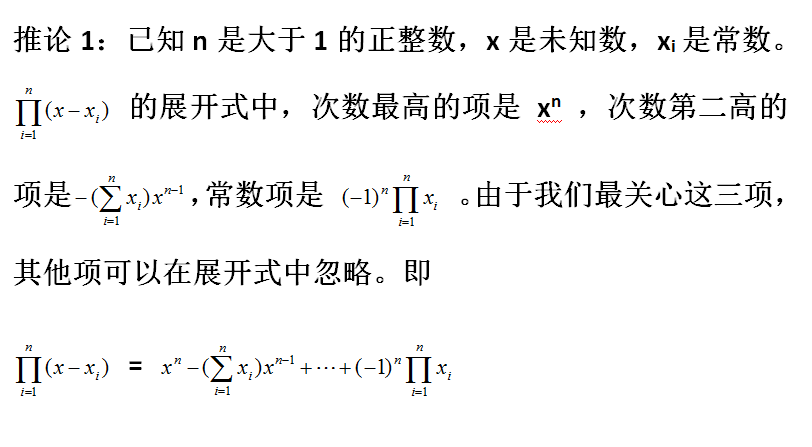证明：

用数学归纳法证明。

当n=2，(x - x1)(x - x2) = x2 - (x1 + x2)x + (-1)2x1x2 命题成立。

当n=3，(x - x1)(x - x2)(x - x3) = (x - x3)[x2 - (x1 + x2)x + (-1)2x1x2]

= x[x2 - (x1 + x2)x + (-1)2x1x2] + (-x3)[x2 - (x1 + x2)x + (-1)2x1x2]

= x3 - (x1 + x2)x2 + x1x2x + (- x3)x2 + (x1 + x2)x3x - x1x2x3

= x3 - (x1 + x2 + x3)x2 + (x1x2 + x1x3 + x2x3)x + (-1)3 x1x2x3

命题成立。

当n=k，假设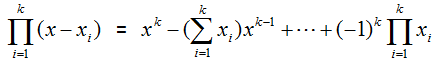当n=k+1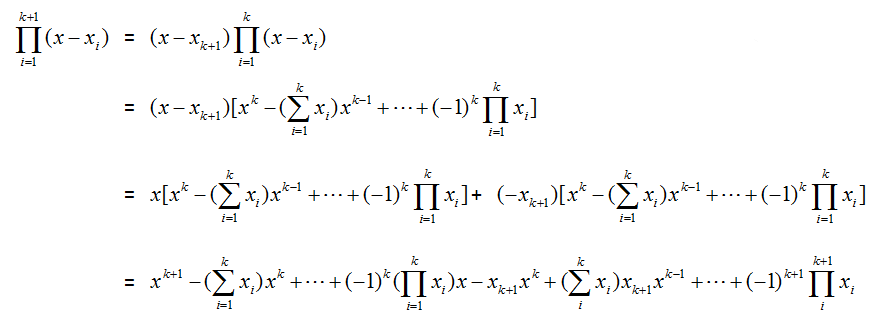因为我们只关心次数最高的项、次数第二高的项和常数项，所以把上面的式子合并同类项后，可以像下面这样简略表示：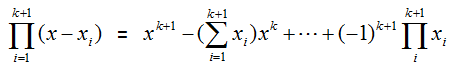综上所述可以证明推论1 。

## 正文

### 复数范围内，如果一元n次方程 anxn+an-1xn-1+···+a0 = 0 的解是 x1, x2, ···, xn。那么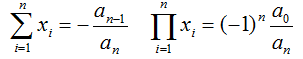证明：

代数基本定理：任何复系数一元n次多项式 方程在复数域上至少有一根(n≥1)，由此推出，n次复系数多项式方程在复数域内有且只有n个根（重根按重数计算）。

已知这些解是x1, x2, ···, xn 。那么一元n次方程可以化成如下形式：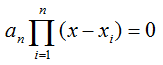根据推论1: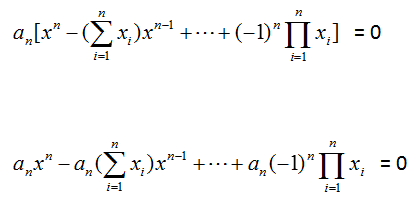所以xn-1 项系数：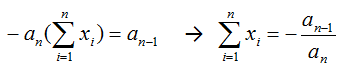常数项：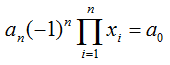若n是偶数，则(-1)n =1，等式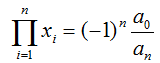成立。

若n是奇数，则(-1)n =-1，等式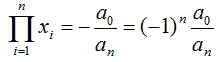成立。

所以综上所述题目得证。

展开全文数学
•文档
•文档
• 一元二次方程判别式与韦达定理完美概要PPT学习教案.pptx
• 一元二次方程的解法(十字交叉法)及韦达定理.pdf
• 一元二次方程根的判别式及韦达定理常见题型及注意事项.doc文档
• 一元二次方程根与系数的关系(韦达定理)判别式与系数必考考点练习题.pdf...